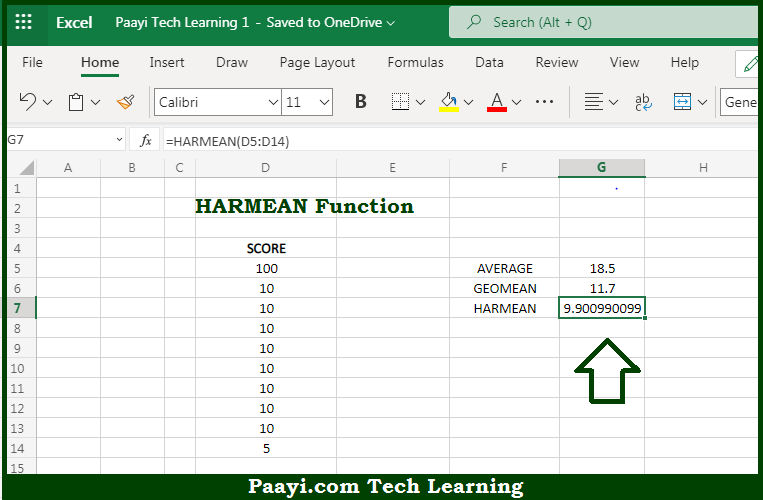# Learn How to Use Microsoft Excel HARMEAN Function

Written by | 0 Comments | 633 Views

In this article, you will learn how to use the Microsoft Excel HARMEAN function and its prime function in Microsoft Excel. You will also get to know the Microsoft Excel HARMEAN function return value and syntax with the help of some examples.

Microsoft Excel HARMEAN Function

The main purpose of the Microsoft Excel HARMEAN function is to calculate the harmonic mean. That implies, with the help of HARMEAN function you can able to return the harmonic mean for a set of numeric values. It should be noted that the harmonic mean is the reciprocal of the arithmetic mean of reciprocals. The Harmonic mean can be used to calculate a mean that reduces the impact of outliers. So, with the help of HARMEAN function, you can able to calculate the harmonic mean for a set of numeric values.

Return Value of HARMEAN Function

The return value will be the calculated mean.

Syntax of HARMEAN Function

=HARMEAN(number1, [number2], ...)

Where the arguments:

• number1: This is the first value or reference.
• number2: This is the second value or reference (optional).

## How to Use Microsoft Excel HARMEAN Function?So we know that Microsoft Excel HARMEAN function you can able to calculate the harmonic mean. That implies, with the help of HARMEAN function you can able to return the harmonic mean for a set of numeric values. It should be noted that the harmonic mean is the reciprocal of the arithmetic mean of reciprocals. The Harmonic mean can be used to calculate a mean that reduces the impact of outliers. So, with the help of HARMEAN function, you can able to calculate the harmonic mean for a set of numeric values.